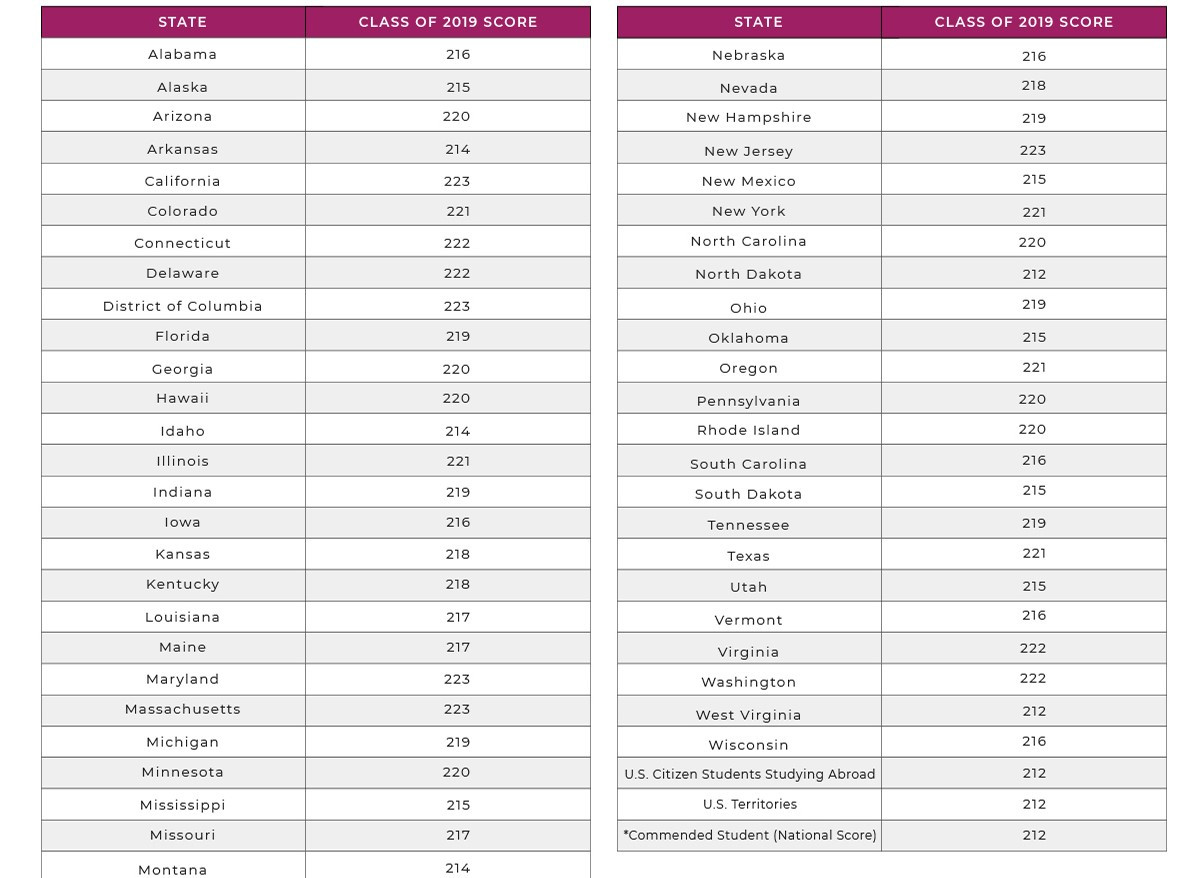# How is the PSAT Scored?One question we often get is: How is the PSAT Scored?

The scoring of the PSAT has mystified parents and students nationwide. So let us make the wait for those national merit scholarship scores a bit less stressful.

Let’s break it down.

### Why Isn’t the National Merit Score on the 1520 scale?

A confusion we often hear is “Why isn’t the National Merit score something like 1400?”

Well, it is and it isn’t. So technically, it’s not a number like 1500. But the index score is actually the same as the PSAT score itself.

The College Board uses the index score as the “starting mark” and then multiplies it to get the final PSAT score.

So to get the PSAT score from the index score:

2. With math you double it and then multiply it by 10.

You might be wondering: how do they get the index score? The index score just goes through a conversion that SAT does per section on each test and then is doubled. You can usually find the conversion chart on the back of most test booklets or in the packet you get back from the College Board if you order the test materials back.

#### PSAT Index Score

So for example, let’s say a student got these following section scores, based on how many questions they got right

Reading (36), Writing (36), Math (38). Index score: (36 + 36 + 38) x 2 = 220

#### PSAT Final Score

Reading + Writing x 10: 36 + 36 = 72
72 x 10 = 720 composite

Math x 2 x10: 38 x 2 x 10= 760 composite (perfect math score)

720 + 760 = 1480 in this case.

Thus, it’s the same score whether it’s in “index” form or on the 1520 scale. But that’s just not how the score will be presented, so we just don’t want parents or students to be confused — but it’s the exact same score, just presented before it’s converted to the 1520 scale.

### What Are the National Merit Cutoff Scores for the Class of 2019?We hope that helps to clear up any confusion about “How is the PSAT scored?” and National Merit! If you’re interested in doing test prep for your student, be sure to check out our offerings!